# Arithmetic Sequences

An arithmetic sequence is an ordered list – usually of numbers – such that there is a common difference between terms.

Here is an example:Here is a second example:Notice that in each of these examples, the common difference between consecutive terms is 3.

# Fill in the gaps

The following applets generate arithmetic sequences. Use any method to calculate the missing numbers.

# Notation

When describing arithmetic sequences, we generally use the following letters:

•refers to the place value (refers to the first place.)
•refers to the term in theplace** (refers to the third term.)
•is often used in place of, the first term.
•is generally used to refer to the common difference.

*Note that,, and other letters are also commonly used for the terms of sequences.

Use these letters to generalise the calculations you used in each of the first two applets.

# The General Term

Example:Substitute,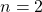,, etc to calculate the first few terms of the sequence.This general term generates the sequence.

You may notice that the general term has the same structure as the equation of a straight line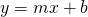, where instead of variablesand, we haveand.

# Calculate the General Term

There are several methods that can be used. Here is one.

This method requires that you already know the value of the first termand the value of the common difference.

In the applet below, you are given the first term, and the common difference. Calculate the term in the blue box.

Calling the first termand the common difference, generalise the calculation for

1.2.3.The answer to the third question is a formula that may be used to calculate the general term for an arithmetic sequence.

## Example 1

An arithmetic sequence has first termand common difference. Find an expression for any term of the sequence,.

Solution

The first five terms of the sequence areWe know that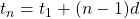. We can replacewithand we can replacewith.It’s helpful to put the 3 before the brackets:We can conclude that.

Notice that in the general term, the common differenceis the coefficient of. That is,, whereremains to be calculated.

Now we can use this formula to find any term in the sequence: let’s find the fifth term. We replace the valuewith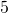..

We can confirm this calculation with the sequence written out above.

## Example 2

An arithmetic sequence has first termand common difference. Find an expression for any term of the sequence,.

Solution

The first five terms of the sequence areWe know that. We can replacewithand we can replacewith.It’s helpful to put the (-3) before the brackets, remember to take the negative sign with you:We can conclude that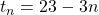.

# The General Term given Any Two Terms

In the figure below we are given two terms of an arithmetic sequence, namelyand.To find the common difference, first note that.

There are four ‘steps’ betweenand. So we divide the difference into four.The common difference between consecutive terms is.

We can find the common difference using a calculation like this (in essence, the ‘slope’ formula):Since the common difference is 9, we know that the general term is, whereremains to be calculated.

To calculate, let’s substitute one of our known terms:. Here, the term is 16 and it is in the third place, so.We can check this expression with the other known term. Let’s make sure that this expression gives us 52 for the seventh term.# Practice

(Note thatis used in place ofin this problem set.